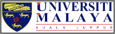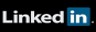# About the Journal

### Hyperscience International Journal is an International Open Access Journal with OJS (Open Journal Systems) publishing quarterly and covering all parts of Sciences in Mathematics (and related), Theoretical & Experimental Physics, Astronomy, Astrophysics, Engineering (in all fields such as Mechanic), Computer Science, Data Science, Geology and Geophysics, GIS, IT, etc.

Mathematics is considered the science of number, quantity, and space. It can either be abstract concepts or concepts applied to other disciplines, such as Physics, Engineering, and Computer science.
In physics, mathematics has been described as "an essential tool", used to prove theories and justify hypotheses made by physicists. Mathematics can also be defined as a natural numerical analysis, whereas the product that we obtain from those analyses is called engineering (from mechanical to industrial.)
Different subfields in mathematics, such as Logic and Linear algebra, can also be recognized as the foundation of other fields, such as computer science. Computer science can be described as the study of computers and computational systems, and it sometimes can even be viewed as a mathematical science due to its close relationship with mathematics.Astronomy & Astrophysics Data Center

-----------------------------------------------------------------------

### The following Data Collected From PHL (Planetary Habitability Laboratory)

This is a collection of websites with data related to planetary habitability studies of astrobiology in general. Many have their own visualization tools or check the Software Tools section for suggestions.